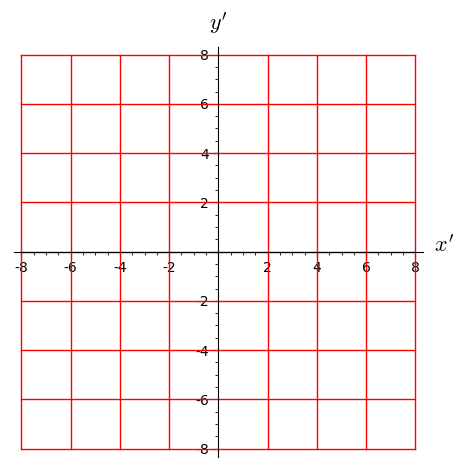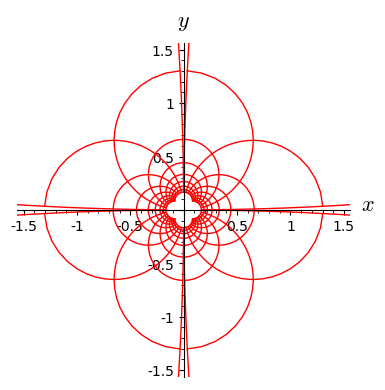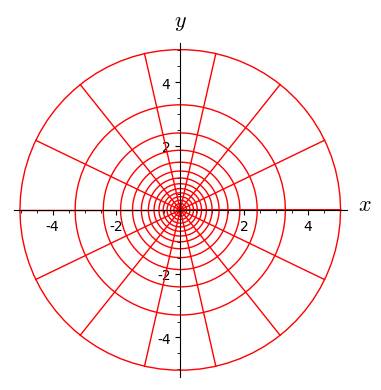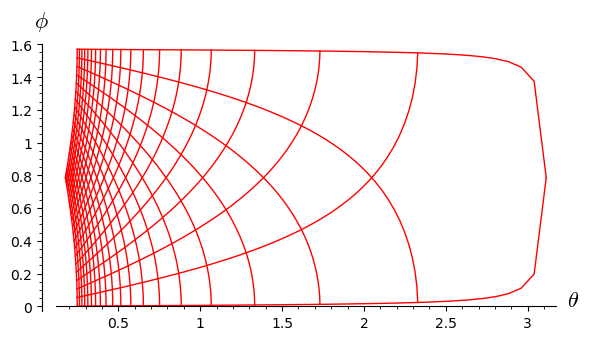# Sphere $\mathbb{S}^2$ (SymPy version)¶

This notebook demonstrates a few differential geometry capabilities of SageMath on the example of the 2-dimensional sphere, using SymPy as symbolic engine, instead of SageMath's default one (Pynac+Maxima). The relevent tools have been implemented through the SageManifolds project.

Click here to download the notebook file (ipynb format). To run it, you must start SageMath with the Jupyter interface, via the command sage -n jupyter

NB: a version of SageMath at least equal to 8.3 is required to run this worksheet:

In :
version()

Out:
'SageMath version 8.8, Release Date: 2019-06-26'

First we set up the notebook to display mathematical objects using LaTeX rendering:

In :
%display latex
from sympy import init_printing
init_printing()


We also define a viewer for 3D plots (use 'threejs' or 'jmol' for interactive 3D graphics):

In :
viewer3D = 'threejs' # must be 'threejs', jmol', 'tachyon' or None (default)


## $\mathbb{S}^2$ as a 2-dimensional differentiable manifold¶

We start by declaring $\mathbb{S}^2$ as a differentiable manifold of dimension 2 over $\mathbb{R}$:

In :
S2 = Manifold(2, 'S^2', latex_name=r'\mathbb{S}^2', start_index=1)


The first argument, 2, is the dimension of the manifold, while the second argument is the symbol used to label the manifold.

The argument start_index sets the index range to be used on the manifold for labelling components w.r.t. a basis or a frame: start_index=1 corresponds to $\{1,2\}$; the default value is start_index=0 and yields to $\{0,1\}$.

In :
print(S2)

2-dimensional differentiable manifold S^2

In :
S2

Out:

The manifold is a Parent object:

In :
isinstance(S2, Parent)

Out:

in the category of smooth manifolds over $\mathbb{R}$:

In :
S2.category()

Out:

We ask for all symbolic computations to be performed with SymPy, instead of SageMath's default symbolic engine (Pynac+Maxima, implemented via SageMath's Symbolic Ring):

In :
S2.set_calculus_method('sympy')


### Coordinate charts on $\mathbb{S}^2$¶

The sphere cannot be covered by a single chart. At least two charts are necessary, for instance the charts associated with the stereographic projections from the North pole and the South pole respectively. Let us introduce the open subsets covered by these two charts: $$U := \mathbb{S}^2\setminus\{N\},$$  $$V := \mathbb{S}^2\setminus\{S\},$$ where $N$ is a point of $\mathbb{S}^2$, which we shall call the North pole, and $S$ is the point of $U$ of stereographic coordinates $(0,0)$, which we call the South pole:

In :
U = S2.open_subset('U') ; print(U)

Open subset U of the 2-dimensional differentiable manifold S^2

In :
V = S2.open_subset('V') ; print(V)

Open subset V of the 2-dimensional differentiable manifold S^2


We declare that $\mathbb{S}^2 = U \cup V$:

In :
S2.declare_union(U, V)


Then we declare the stereographic chart on $U$, denoting by $(x,y)$ the coordinates resulting from the stereographic projection from the North pole:

In :
stereoN.<x,y> = U.chart()


The expression .<x,y> in the left-hand side means that the Python variables x and y are set to the two coordinates of the chart. This allows one to refer subsequently to the coordinates by their names. In the present case, the function chart() has no argument, which implies that the coordinate symbols will be x and y (i.e. exactly the characters appearing in the <...> operator) and that each coordinate range is $(-\infty,+\infty)$. As we will see below, for other cases, an argument must be passed to chart() to specify each coordinate symbol and range, as well as some specific LaTeX symbol.

In :
stereoN

Out:

The coordinates can be accessed individually, either by means of their indices in the chart ( following the convention start_index=1 set in the manifold's definition) or by their names as Python variables:

In :
stereoN

Out:
In :
y is stereoN

Out:

Similarly, we introduce on $V$ the coordinates $(x',y')$ corresponding to the stereographic projection from the South pole:

In :
stereoS.<xp,yp> = V.chart("xp:x' yp:y'")


In this case, the string argument passed to chart stipulates that the text-only names of the coordinates are xp and yp (same as the Python variables names defined within the <...> operator in the left-hand side), while their LaTeX names are $x'$ and $y'$.

In :
stereoS

Out:

At this stage, the user's atlas on the manifold has two charts:

In :
S2.atlas()

Out:

We have to specify the transition map between the charts 'stereoN' = $(U,(x,y))$ and 'stereoS' = $(V,(x',y'))$; it is given by the standard inversion formulas:

In :
stereoN_to_S = stereoN.transition_map(stereoS,
(x/(x^2+y^2), y/(x^2+y^2)),
intersection_name='W',
restrictions1= x^2+y^2!=0,
restrictions2= xp^2+xp^2!=0)
stereoN_to_S.display()

Out:

In the above declaration, 'W' is the name given to the chart-overlap subset: $W := U\cap V$, the condition $x^2+y^2 \not=0$  defines $W$ as a subset of $U$, and the condition $x'^2+y'^2\not=0$ defines $W$ as a subset of $V$.

The inverse coordinate transformation is computed by means of the method inverse():

In :
stereoS_to_N = stereoN_to_S.inverse()
stereoS_to_N.display()

Out:

In the present case, the situation is of course perfectly symmetric regarding the coordinates $(x,y)$ and $(x',y')$.

At this stage, the user's atlas has four charts:

In :
S2.atlas()

Out:

Let us store $W = U\cap V$ into a Python variable for future use:

In :
W = U.intersection(V)


Similarly we store the charts $(W,(x,y))$ (the restriction of  $(U,(x,y))$ to $W$) and $(W,(x',y'))$ (the restriction of $(V,(x',y'))$ to $W$) into Python variables:

In :
stereoN_W = stereoN.restrict(W)
stereoN_W

Out:
In :
stereoS_W = stereoS.restrict(W)
stereoS_W

Out:

We may plot the chart $(W, (x',y'))$ in terms of itself, as a grid:

In :
stereoS_W.plot()

Out:More interestingly, let us plot the stereographic chart $(x',y')$ in terms of the stereographic chart $(x,y)$ on the domain $W$ where both systems overlap (we split the plot in four parts to avoid the singularity at $(x',y')=(0,0)$):

In :
graphSN1 = stereoS_W.plot(stereoN, ranges={xp:[-6,-0.02], yp:[-6,-0.02]})
graphSN2 = stereoS_W.plot(stereoN, ranges={xp:[-6,-0.02], yp:[0.02,6]})
graphSN3 = stereoS_W.plot(stereoN, ranges={xp:[0.02,6], yp:[-6,-0.02]})
graphSN4 = stereoS_W.plot(stereoN, ranges={xp:[0.02,6], yp:[0.02,6]})
show(graphSN1+graphSN2+graphSN3+graphSN4,
xmin=-1.5, xmax=1.5, ymin=-1.5, ymax=1.5)### Spherical coordinates

The standard spherical (or polar) coordinates $(\theta,\phi)$ are defined on the open domain $A\subset W \subset \mathbb{S}^2$ that is the complement of the "origin meridian"; since the latter is the half-circle defined by $y=0$ and $x\geq 0$, we declare:

In :
A = W.open_subset('A', coord_def={stereoN_W: (y!=0, x<0),
stereoS_W: (yp!=0, xp<0)})
print(A)

Open subset A of the 2-dimensional differentiable manifold S^2


The restriction of the stereographic chart from the North pole to $A$ is

In :
stereoN_A = stereoN_W.restrict(A)
stereoN_A

Out:

We then declare the chart $(A,(\theta,\phi))$ by specifying the intervals $(0,\pi)$ and $(0,2\pi)$ spanned by respectively $\theta$ and $\phi$:

In :
spher.<th,ph> = A.chart(r'theta:(0,pi):\theta phi:(0,2*pi):\phi') ; spher

Out:

The specification of the spherical coordinates is completed by providing the transition map with the stereographic chart $(A,(x,y))$:

In :
spher_to_stereoN = spher.transition_map(stereoN_A,
(sin(th)*cos(ph)/(1-cos(th)),
sin(th)*sin(ph)/(1-cos(th))))
spher_to_stereoN.display()

Out:

We also provide the inverse transition map, asking to check that the provided formulas are indeed correct (argument verbose=True):

In :
spher_to_stereoN.set_inverse(2*atan(1/sqrt(x^2+y^2)), atan2(-y,-x)+pi,
verbose=True)

Check of the inverse coordinate transformation:
theta == 2*arctan(sqrt(-cos(theta) + 1)/sqrt(cos(theta) + 1))
phi == pi + arctan2(sin(phi)*sin(theta)/(cos(theta) - 1), cos(phi)*sin(theta)/(cos(theta) - 1))
x == x
y == y


The check is passed, modulo some lack of trigonometric simplifications in the first two lines.

In :
spher_to_stereoN.inverse().display()

Out:

The transition map $(A,(\theta,\phi))\rightarrow (A,(x',y'))$ is obtained by combining the transition maps $(A,(\theta,\phi))\rightarrow (A,(x,y))$ and $(A,(x,y))\rightarrow (A,(x',y'))$:

In :
stereoN_to_S_A = stereoN_to_S.restrict(A)
spher_to_stereoS = stereoN_to_S_A * spher_to_stereoN
spher_to_stereoS.display()

Out:

Similarly, the transition map $(A,(x',y'))\rightarrow (A,(\theta,\phi))$ is obtained by combining the transition maps $(A,(x',y'))\rightarrow (A,(x,y))$ and $(A,(x,y))\rightarrow (A,(\theta,\phi))$:

In :
stereoS_to_N_A = stereoN_to_S.inverse().restrict(A)
stereoS_to_spher = spher_to_stereoN.inverse() * stereoS_to_N_A
stereoS_to_spher.display()

Out:

The user atlas of $\mathbb{S}^2$ is now

In :
S2.atlas()

Out:

Let us draw the grid of spherical coordinates $(\theta,\phi)$ in terms of stereographic coordinates from the North pole $(x,y)$:

In :
spher.plot(stereoN, number_values=15, ranges={th: (pi/8,pi)})

Out:Conversly, we may represent the grid of the stereographic coordinates $(x,y)$ restricted to $A$ in terms of the spherical coordinates $(\theta,\phi)$. We limit ourselves to one quarter (cf. the argument ranges):

In :
stereoN_A.plot(spher, ranges={x: (0.01,8), y: (0.01,8)}, number_values=20, plot_points=200)

Out:## Points on $\mathbb{S}^2$

We declare the North pole (resp. the South pole) as the point of coordinates $(0,0)$ in the chart $(V,(x',y'))$ (resp. in the chart $(U,(x,y))$):

In :
N = V.point((0,0), chart=stereoS, name='N') ; print(N)
S = U.point((0,0), chart=stereoN, name='S') ; print(S)

Point N on the 2-dimensional differentiable manifold S^2
Point S on the 2-dimensional differentiable manifold S^2


Since points are Sage Element's, the corresponding Parent being the manifold subsets, an equivalent writing of the above declarations is

In :
N = V((0,0), chart=stereoS, name='N') ; print(N)
S = U((0,0), chart=stereoN, name='S') ; print(S)

Point N on the 2-dimensional differentiable manifold S^2
Point S on the 2-dimensional differentiable manifold S^2


Moreover, since stereoS in the default chart on $V$ and stereoN is the default one on $U$, their mentions can be omitted, so that the above can be shortened to

In :
N = V((0,0), name='N') ; print(N)
S = U((0,0), name='S') ; print(S)

Point N on the 2-dimensional differentiable manifold S^2
Point S on the 2-dimensional differentiable manifold S^2

In :
N.parent()

Out:
In :
S.parent()

Out:

We have of course

In :
N in V

Out:
In :
N in S2

Out:
In :
N in U

Out:
In :
N in A

Out:

Let us introduce some point at the equator:

In :
E = S2((0,1), chart=stereoN, name='E')


The point $E$ is in the open subset $A$:

In :
E in A

Out:

We may then ask for its spherical coordinates $(\theta,\phi)$:

In :
E.coord(spher)

Out:

which is not possible for the point $N$:

In :
try:
N.coord(spher)
except ValueError as exc:
print('Error: ' + str(exc))

Error: the point does not belong to the domain of Chart (A, (theta, phi))


## Mappings between manifolds: the embedding of $\mathbb{S}^2$ into $\mathbb{R}^3$

Let us first declare $\mathbb{R}^3$ as a 3-dimensional manifold covered by a single chart (the so-called Cartesian coordinates):

In :
R3 = Manifold(3, 'R^3', r'\mathbb{R}^3', start_index=1)
R3.set_calculus_method('sympy')
cart.<X,Y,Z> = R3.chart() ; cart

Out:

The embedding of the sphere is defined as a differential mapping $\Phi: \mathbb{S}^2 \rightarrow \mathbb{R}^3$:

In :
Phi = S2.diff_map(R3, {(stereoN, cart):
[2*x/(1+x^2+y^2), 2*y/(1+x^2+y^2),
(x^2+y^2-1)/(1+x^2+y^2)],
(stereoS, cart):
[2*xp/(1+xp^2+yp^2), 2*yp/(1+xp^2+yp^2),
(1-xp^2-yp^2)/(1+xp^2+yp^2)]},
name='Phi', latex_name=r'\Phi')

In :
Phi.display()

Out:
In :
Phi.parent()

Out:
In :
print(Phi.parent())

Set of Morphisms from 2-dimensional differentiable manifold S^2 to 3-dimensional differentiable manifold R^3 in Category of smooth manifolds over Real Field with 53 bits of precision

In :
Phi.parent() is Hom(S2, R3)

Out:

$\Phi$ maps points of $\mathbb{S}^2$ to points of $\mathbb{R}^3$:

In :
N1 = Phi(N) ; print(N1) ; N1 ; N1.coord()

Point Phi(N) on the 3-dimensional differentiable manifold R^3

Out:
In :
S1 = Phi(S) ; print(S1) ; S1 ; S1.coord()

Point Phi(S) on the 3-dimensional differentiable manifold R^3

Out:
In :
E1 = Phi(E) ; print(E1) ; E1 ; E1.coord()

Point Phi(E) on the 3-dimensional differentiable manifold R^3

Out:

$\Phi$ has been defined in terms of the stereographic charts $(U,(x,y))$ and $(V,(x',y'))$, but we may ask its expression in terms of spherical coordinates. The latter is then computed by means of the transition map $(A,(x,y))\rightarrow (A,(\theta,\phi))$:

In :
Phi.expr(stereoN_A, cart)

Out:
In :
Phi.expr(spher, cart)

Out:
In :
Phi.display(spher, cart)

Out:

Let us use $\Phi$ to draw the grid of spherical coordinates $(\theta,\phi)$ in terms of the Cartesian coordinates $(X,Y,Z)$ of $\mathbb{R}^3$:

In :
graph_spher = spher.plot(chart=cart, mapping=Phi, number_values=11,
color='blue', label_axes=False)
show(graph_spher, viewer=viewer3D)


We may also use the embedding $\Phi$ to display the stereographic coordinate grid in terms of the Cartesian coordinates in $\mathbb{R}^3$. First for the stereographic coordinates from the North pole:

In :
graph_stereoN = stereoN.plot(chart=cart, mapping=Phi, number_values=25,
label_axes=False)
show(graph_stereoN, viewer=viewer3D)


and then have a view with the stereographic coordinates from the South pole superposed (in green):

In :
graph_stereoS = stereoS.plot(chart=cart, mapping=Phi, number_values=25,
color='green', label_axes=False)
show(graph_stereoN + graph_stereoS, viewer=viewer3D)